CAT  >  Test Level 3: Exponents and Logarithm

# Test Level 3: Exponents and Logarithm

Test Description

## 10 Questions MCQ Test Level-wise Tests for CAT | Test Level 3: Exponents and Logarithm

Test Level 3: Exponents and Logarithm for CAT 2023 is part of Level-wise Tests for CAT preparation. The Test Level 3: Exponents and Logarithm questions and answers have been prepared according to the CAT exam syllabus.The Test Level 3: Exponents and Logarithm MCQs are made for CAT 2023 Exam. Find important definitions, questions, notes, meanings, examples, exercises, MCQs and online tests for Test Level 3: Exponents and Logarithm below.
Solutions of Test Level 3: Exponents and Logarithm questions in English are available as part of our Level-wise Tests for CAT for CAT & Test Level 3: Exponents and Logarithm solutions in Hindi for Level-wise Tests for CAT course. Download more important topics, notes, lectures and mock test series for CAT Exam by signing up for free. Attempt Test Level 3: Exponents and Logarithm | 10 questions in 20 minutes | Mock test for CAT preparation | Free important questions MCQ to study Level-wise Tests for CAT for CAT Exam | Download free PDF with solutions
 1 Crore+ students have signed up on EduRev. Have you?
Test Level 3: Exponents and Logarithm - Question 1

### What is the value of x + y in the following two equations?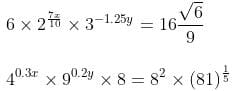Detailed Solution for Test Level 3: Exponents and Logarithm - Question 1

The given equations in the simplest form can be written as: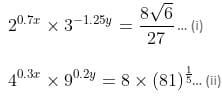From equation (ii),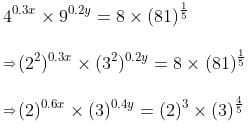⇒ 0.6x = 3
⇒ x = 5
And 0.4y = 4/5
⇒ y = 2
So, the sum of the values of x and y is given as 7.

Test Level 3: Exponents and Logarithm - Question 2

### 1 + loge z +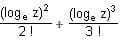+ ... is equal to

Detailed Solution for Test Level 3: Exponents and Logarithm - Question 2

We know that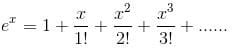Put x = loge(z)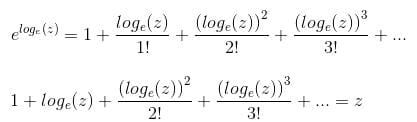Test Level 3: Exponents and Logarithm - Question 3

### The population of microbial bacteria "Gram staminee" increases exponentially as P(t) = aebt. The population of bacteria at the beginning of experiment at 10 a.m. was 20, which grows up to 40 by 12 p.m. What will be the population of bacteria at 3 p.m.?

Detailed Solution for Test Level 3: Exponents and Logarithm - Question 3

Initially at 10 a.m., i.e. at t = 0, population of the bacteria was 20.
At 12 p.m., t = 2 and at 3 p.m., t = 5
Now, P(0) = aeb(0) = ae0 = a = 20 (given)
Now, at t = 2, P(t) = 40
⇒ 40 = 20eb(2)
⇒ e2b = 2
Taking log of both sides, we get
2b = loge 2 (log e = 1)
2b = 0.693
b =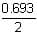= 0.346
∴ P(t) = 20e0.346t
∴ P(5) = 20e0.346 x 5
= 20 x 5.64
= 112.8
Hence, population of bacteria at 3 p.m. = 113

Test Level 3: Exponents and Logarithm - Question 4

If 10x = x50, find the value of x.

Detailed Solution for Test Level 3: Exponents and Logarithm - Question 4

10x = x50
Take log of both L.H.S. and R.H.S.
L.H.S. = log (10x) = x (log 10) = x (because log 10 = 1)
R.H.S = log (x50) = 50 log x
L.H.S. = R.H.S.
x = 50 log x

Case I:
If x = 1, then
1 = 50 (log 1) (because log 1 = 0)
1 = 0 (Not possible)

Case II:
If x = 10, then
10 = 50 log (10)
10 = 50 (Not possible) (because log 10 = 1)

Case III:
If x = √10, then
√10= 50 log (√10)
√10 = 25 (Not possible) (because log √10 = 1/2)

Case IV:
If x = √10, then
√10 = 50 log (100) = 50 log [(10)2]
= 50 × 2 log (10) (because log a = x log a)
= 50 × 2 × 1 (because log 10 = 1)
100 = 100
L.H.S. = R.H.S.
Hence, x = 100

Test Level 3: Exponents and Logarithm - Question 5

If P = log23 and Q = log69, which of the following options is true?

Detailed Solution for Test Level 3: Exponents and Logarithm - Question 5

log22 < log23 < log24
i.e. 1 < P < 2
Similarly, log66 < log69 < log636
1 < Q < 2
Both P and Q belong to the interval (1, 2).
We shall now compare both P and Q with the average of 1 and 2, i.e. 3/2.
Let us assume that log2 3 > 3/2
Then 3 > 23/2 ⇒ 32 > 23
i.e. 9 > 8. This is true
P > 3/2 is correct.
We shall also suppose that log6 9 > 3/2
i.e. 9 > 63/2
92 > 63
i.e. 81 > 216 is not true
Hence Q > 3/2 is not correct.
Q < 3/2
Therefore P > Q.

Test Level 3: Exponents and Logarithm - Question 6

If f(x) =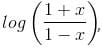then f(x) + f(y) is equal to

Detailed Solution for Test Level 3: Exponents and Logarithm - Question 6

f(x) + f(y) =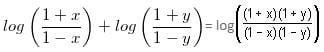f(x) + f(y) = log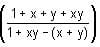f(x) + f(y) = log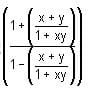f(x) + f(y) = f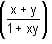Test Level 3: Exponents and Logarithm - Question 7

If 46 + 12 + 18 + 24 + … + 6x = (0.0625)-84, then what is the value of x?

Detailed Solution for Test Level 3: Exponents and Logarithm - Question 7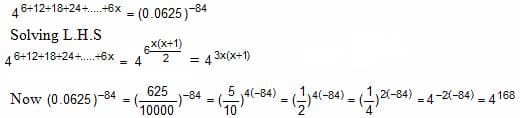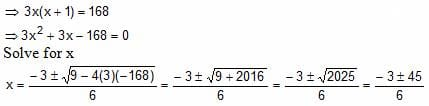Thus, x = 7, -8
Since x cannot be negative value, x = 7 is the answer.

Test Level 3: Exponents and Logarithm - Question 8

The radioactive decay of a radioactive element is given as per the exponential function A(t) = A0 × eat, where t is half-life of that element and A0 is the amount of the element present initially. In a disastrous nuclear leakage incident in Chernobyl, in 1992, the level of plutonium-80 was found to be 20 times the safe level. If the half-life of plutonium-80 is 20 years, how long will it take for plutonium-80 level to reach the safe limits?

Detailed Solution for Test Level 3: Exponents and Logarithm - Question 8

The radioactive decay is given as per the exponential function A(t) = A0 × eat.
The half-life of plutonium-80 is 20 years.
After 20 years, the amount of plutonium-80 left will be half.
∴ 0.5 A0 = A0 × ea × 20
0.5 = ea × 20
On taking log of both sides, the equation becomes:
loge (0.5) = a × 20 (log e = 1)
a = 1/20 (loge 0.5)
= -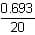= -0.0346
As it is given, after 20 years, radioactivity is reduced to one-twentieth of the initial activity.Hence, A(t) = 1/20 A0
Substituting this in the activity equation, we get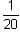A0 = A0 e-0.346t
0.05 = e-0.346t
On taking log of both the sides, the equation becomes:
loge (0.05) = -0.0346t
-2.9957 = -0.346 t
t =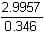= 8.66 years

Test Level 3: Exponents and Logarithm - Question 9

Find the value of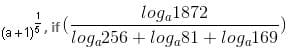loga961 = 1.

Detailed Solution for Test Level 3: Exponents and Logarithm - Question 9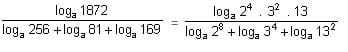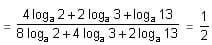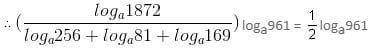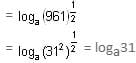Now, loga31 = 1
a = 31
So, (a + 1) = 32
(a + 1)(1/5) = 32(1/5) = 2

Test Level 3: Exponents and Logarithm - Question 10

The value of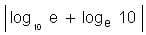is

Detailed Solution for Test Level 3: Exponents and Logarithm - Question 10

|log10 e + loge 10|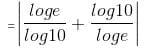If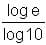= x, then the given expression can be written as: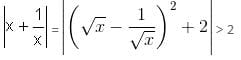Hence, the value of the given expression is always greater than 2.

## Level-wise Tests for CAT

5 docs|272 tests
 Use Code STAYHOME200 and get INR 200 additional OFF Use Coupon Code
Information about Test Level 3: Exponents and Logarithm Page
In this test you can find the Exam questions for Test Level 3: Exponents and Logarithm solved & explained in the simplest way possible. Besides giving Questions and answers for Test Level 3: Exponents and Logarithm, EduRev gives you an ample number of Online tests for practice

## Level-wise Tests for CAT

5 docs|272 tests

### How to Prepare for CAT

Read our guide to prepare for CAT which is created by Toppers & the best Teachers